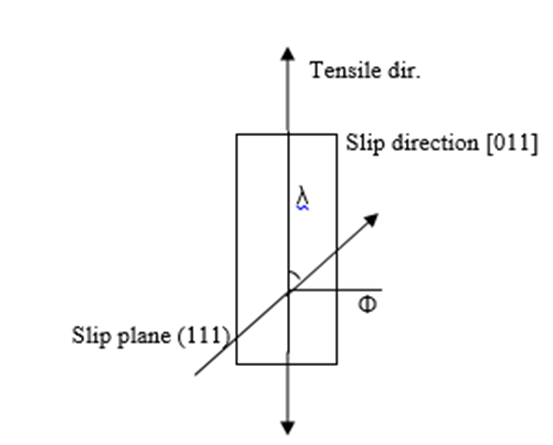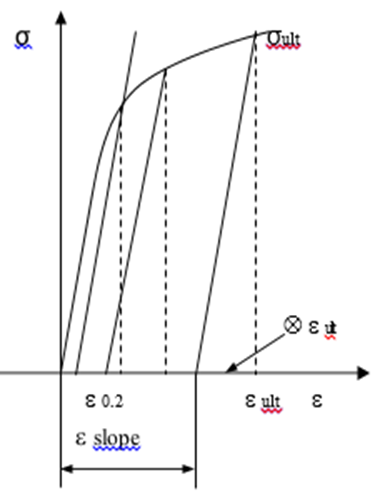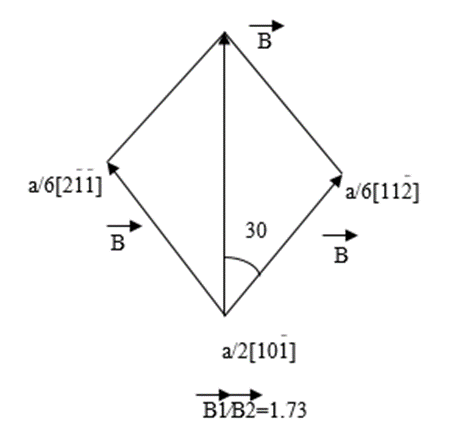### The Dislocation and Twins on Deformation Behaviours by Calculating DS in -Tial

###### Journal Name : SunText Review of Material Science

Article Type : Research Article

Authors : Xu R, Anh HJ, Yong HB and Kim YW

Keywords : Dislocation & Twin; Directional solidify (DS); Deformation; Schmid factor; Orientation; TiAl; Calculating

### Abstract

The m of 0.167 in the rate of 1.25E-02 was higher than 0.09 in the rate of 1.25E in the twins of {011} <111(—)]. The LnK will change to 6.31 correspondingly in terms of the decreased strain rate. They had shown that low strain rate cause high m. In the case of 22? they might approach virtual Schmid factor 0.356 while the 0.524 of 11? was gained in {111(—)} <011(—)]. The low Schmid factor was caused under 22?. Moreover Frank partial dislocation was easily formed than Shockley partial one due to its low value. That Frank partial dislocation will be formed as many as possible was mainly emphasized in TiAl material. On the other side Shockley partial one was hard formed. Unit one will stand between them to play a certain role in the material.

### Introduction

TiAl alloys have had high strength and high temperature strength compared with other high temperature (HT) alloys. Anti-oxidized and better creep properties was dominant as very promising material to be substituted for Ti and Ni base. In particular DS TiAl alloys with an aligned lamellar microstructure (MS) have a very good combination of strength and ductility over a wide temperature range . The twin direction could be aligned along symmetric shaft. Others may also play role to Schmid factor of DS part. Twins and dislocation was as shown there, detail discussion will be taken on below. Super dislocation was high energy with  and easy slip was low with 1/2. That flow easy slip has depended on Peierls stress method. With basal a slip on prismatic the activated deformation modes in six domains of ? phase respectively. {111}<110] slip by ordinary dislocations, {111} <101] by super dislocations, and {111} <112] with twins. So there were 16 deformation systems in each ? domain. In the present, the twin mechanism was considered as a deformation mode like the other slip modes. However the twin can only be initiated in one direction . For the needs of higher temperature and light quality, new advanced materials would be searched: 1) high melting point; 2) low density; 3) elastic modulus; 4) good structure stability and excellent oxidized resistance in the high temperature application, such as engines [3,4].

### Numerical Procedure

The results was proceeded with the equations of above, and according to Figure 1 the logic arrangement was done (Figure 1). Hence we obtained the series value as below discussed. The deformation behaviour could be computed in terms of mathematical method. The curves of stress and strain may be calculated used on formula as below. The curve was shown in Figure 2 for calculating constant. The calculated method could be described as following typically (Figure 2). The yield stress and ultimate strain may be used to compute. Firstly, Elastic modulus might be based on data. Secondly the plastic deformation could be thought simply as formula. The calculation method had been used to obtain the slope to be measured and confirmed.

s tr= s(1+e), s= E Äe  (1)

?tr=LN(1+e)   (2)

The Critical resolved shear stress (CRSS) had been calculated with method of orientations. As for plastic of DS the tension and compression was used to obtain the equation of stress and strain. The CRSS was formulated with yield stress of shear stress. The Schmid factor was largest slip systems in {111} <110>. The Schmid law had given the CRSS to occur plastic deformation. In the method the c/a of TiAl was used to 1.016. The Schmid factors for possible slip and twinning systems were operated under compression along the c-axis (>0) and approximate with c/a=1.

?c=?*COS(?)*COS(?)   (3)

? was yield stress, ? was angle between tensile axis and slip direction.Figure 1: Schematic of the CRSS of  in (111).Figure 2: Curve of s and e for calculation.Figure 3: The resolved Shockley partial dislocations  from  unit dislocation.

### Result and Discussions

As seen in Table 1 the elastic modulus was decreased to15*cos2 (f) Gpa. The m changes in 0.09 for {111} <011] and {011} <11(—) 1(—)], {111} <112(—)] respectively (Table 1). The of LnK changes in 5.17~5.87 correspondingly. From them the m should approach 0.1 nearly with varied dislocations. As shown in Table 2 when the elastic modulus was 150Gpa the strain rate sensitivity exponent m will increase to 0.091 in the strain rate of 1.25E-02 (Table 2). As the strain rate was high the m was low in {111} <011]. They had shown that low rate cause high m. That would be decreased as the elastic modulus was low to 15GPa. They had shown that high strain rate because low m. That may be another reason. As seen in Table 2 the trend of rate could be found. The m may decrease as both the rate and elastic modulus were low. The m was calculated as follow in Table 1, they were concluded as below. Slight changes were found in m but in K the value was more different than n; that may be concluded as the Schmid factor. But no more difference will be explained for calculated method in particular. There may be varied in terms of main role of slip direction that means we shall pay more attention to the important direction. The low n was the reason for small slope, ie. Small stress raised. The m will be 0.09~0.1 approximately in the whole slips. A little influence on slip. The course would be through that elastic modulus was to multiply square cos? for elastic modulus. As shown in Table 3 the m of 0.167 in the rate of 1.25E-00 was higher than 0.09 in the rate of 1.25E-02 in the {011} <111(—)] while 6.31 of LnC was larger than 5.87 respectively (Table 3). That means larger stress happened on this twins in high strain rate. The condition should be presumed that the  was growth direction, however there might be some direction deviations between the actual growth and . In this paper the same tensile force with the deviated direction that also might be depended on the actual growth direction could play role in specimens. It should be dependent on the actual growth direction could play role in specimens. That also might be depended on the lamellar direction. The variation should fit to the analysis well under vary directions according to the slip systems. As shown in Table 4 in the case of 11° that would have high Schmid factor but in  the  case of 22°  they  might approach virtual Schmid factor 0.356 while the 0.524 of 11° was gained in {111(—)}<011(—)] (Table 4). That cause the low Schmid factor under 22°. The low one of 0.219 to compare with the high 0.424 in {011} <111(—)] indicate the less resistance to plasticity. The {111(—)} <011(—)] that may be higher than the {011} <111(—)].

The highest values was obtained in {111} <112(—)], they were 0.594 in 11° and 0.453 in 22°. The strain sensitivity exponent m was 1.25E-4, and detail course will be in terms of equations. As shown in Table 5 the yield stress of TiAl is 318Mpa for TiAl alloys (Table 5). The elongation of 2.3% was the experimental value with the regulated method which decreased the error causing in the experiment. The E of binary Ti3Al in computation would be 150Gpa for TiAl alloys. The deviation to the reference was very small as 0.001~0.002nm. In Table 5 it showed the constant and physical to compare with reference . The value has been approached approximately. The lattice constants also approached the reference . It showed that values of yield stress was higher than that of binary’s in all the orientations, though the dependence of yield stress was mostly the same as both specimens existent to the compressive axis. The easy type of deformation namely shear deformation parallel to the lamellar boundary occurs when it was tested in orientation with inter mediation angles. So the difference in deformed’ does not exist. Meanwhile the hard type of specimens i.e. shear deforming across the boundaries happens to in both binary and ternary specimens. Hence it was supposed that the promotion of tensile ductility of specimens with orientation B2 had relation to the fractures. The m may decrease as both the rate and elastic modulus were low. As for the parameters in Table 4, the Schmid factor as seen in Figure 1 was checked for the main reason was the mathematical way. Here ?s was the stress, ? was the angle between tensile axis and slip direction. The condition should be presumed that the  was growth direction, however there might be some direction deviations between the actual growth and . In this paper the same tensile force with the deviated direction that also might be depended on the lamellar direction. It should be dependent on the actual growth direction could play role in specimens that also might be depended on the lamellar direction. The lattice constant of c/a has 1.017, on the basis of value that c has 0.407 and a has 0.401nm for g.

a/2?a/3+[112(—)]a/6+SISF ?4?

Here a Frank partial was /3 and Shockley partial was /6 bound a loop. In terms of the calculation of anisotropic elastic line energies, dissociation was feasible as the Shockley partial which was the edge or a mixed character. SISF was the super intrinsic stacking fault energy. It is found that the Frank partial one was easily formed than Shockley partial one from the Table 4 because of its low value.  Then the unit dislocation is between them. The effective forming turn is Frank partial dislocation >unit dislocation >Shockley partial one. That Frank partial dislocation will be formed as many as possible was mainly emphasized in TiAl material.  On the other side Shockley partial one was hard formed. Unit one will stand between them to play a certain role in the material.

a/2[101(—)] ?[112(—)]a/6+[21(—)1(—)]a/6+Fault?5?

a/2[01(—)1]?[12(—)1]a/6+[1(—)1(—)2]a/6+Fault ?6?

The dislocation moving direction were consistent with Burgers vector. The planes of their movement would be existent with Burgers vector and dislocation line. According to plane and directions for movement of atoms that were limited, it was defined that they would have taken place under atomic density with place of mostly high. The yield of material took effect in the atomic structure highly, and the work hardening could be affected by dislocation density. The full dislocation on {001} had been existed in two part resolved dislocations. The true stress and strain in poly crystals like Fcc could be expressed as ?=K ? m. The true stress had one of mechanical features which was sensitive to strain rate. True strain will be increased in terms of high m. with increasing m the true stress increases to enhance deformation rate ?. Under tensile ? was increased so the high elongation will be obtained (Figure 3). As for general metal m was 0.02~0.2. To poly crystals deformation of grain boundary slip will take big role. Under the assumption those states were isothermal, heat generation and heat transfer were not taken into account, plastic deformation by various asymmetric processes [7,8].

### Conclusions

1. The m of 0.167 in the rate of 1.25E-02 was higher than 0.09 in the rate of 1.25E in the twins of {011} <111(—)]. The LnK will change to 6.31 correspondingly in terms of the decreased strain rate. They had shown that low strain rate cause high m.
2. In the case of 22° they might approach virtual Schmid factor 0.356 while the 0.524 of 11° was gained in {111(—)} <011(—)]. That cause the low Schmid factor under 22°. The low one of 0.219 to compare with the high 0.424 in {011} <111(—)] indicate the less resistance to plasticity.
3. The Frank partial dislocation was easily formed than Shockley partial one due to its low value. The effective forming turn was Frank partial dislocation >unit dislocation >Shockley partial one.
4. That Frank partial dislocation will be formed as many as possible was mainly emphasized in TiAl material.  On the other side Shockley partial one was hard formed. Unit one will stand between them to play a certain role in the material.

### References

1. Wang KF, Guo JJ, Fu HZ. Acta Metall. Sini. (Eng. Lett.). 2008; 21: 146.
2. Yoo MH, Hishinuma A. Met. & Mat. 1997; 3: 65.
3. Leyens C, Peters M, Ti & Ti Alloys, WH. 2005; 52-79.
4. Williams JC. Structural Intermetallics. TMS. 1997.
5. Kim YW, Froes FH. HT Aluminids and Intermetallics. TMS. 1990; 465.
6. Run Xu, The relationship of properties with variable mass of block on crank linkage mechanism in multibody system. (American) SunText Rev Mat Sci. 2021.
7. Chun BS, Jung IS. Materials strength and fracture. 1996.
8. Huang SC, Chesnutt JC. Intermetallic Compounds, 2. Practice. 1994; 73.